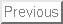Next:Figures

THEORETICAL DESCRIPTIONS OF SHALLOW-WATER SOLITARY
INTERNAL WAVES: COMPARISONS WITH FULLY NONLINEAR WAVES

Kevin G. Lamb

Department of Applied Mathematics, University of Waterloo
Waterloo, Ontario, Canada         N2L 3G1

Two formulations of shallow-water weakly nonlinear theory for internal waves are presented. The structure of solitary waves predicted by these two theories are compared with fully nonlinear solitary waves.

Introduction

In this talk two different formulations of shallow-water, weakly-nonlinear theory will be considered and the predicted structure of solitary waves will be compared with fully nonlinear solitary waves. The latter are computed numerically using an iterative method (1). The two formulations of the theory are obtained by using different sets of independent variables. For the Eulerian formulation the independent variables are (x,z,t) where x and z are the horizontal and vertical Cartesian coordinates and t is time (2,3). For the Euler-Lagrange formulation of the theory z is replaced by the Lagrangian coordinate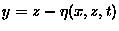where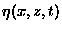is the vertical displacement of the fluid particle at (x,z,t) from its rest position (4).

Eulerian Formulation

Making the incompressible and Boussinesq approximations, neglecting rotation and viscosity, the equations of motion in Cartesian coordinates are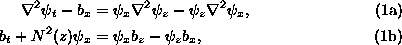where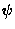is the stream function for the velocity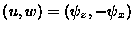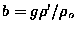where g=9.81 ms-1is the constant gravitational acceleration,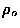is a constant reference density and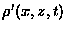is the density perturbation due to the wave, and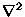is the Laplacian operator. The buoyancy frequency N(z) is given by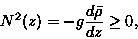(2)

where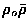is the undisturbed density field. For simplicity it is assumed that there is no background flow. The fluid is assumed to lie between two horizontal, rigid planes at z=0,H so that the boundary conditions are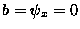at z=0,H.

Under the assumption of small, long waves an asymptotic expansion of the above equations leads to a weakly-nonlinear solution of the form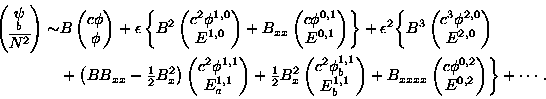(3)

Here B = B(x,t) and the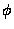and E with the various indices are functions of z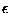is a parameter whose value we take to be one. Nondimensional equations would have the same form, in which caseappears as a small parameter measuring the strength of the nonlinearity and dispersion. Here it is included to indicate the relative ordering of the various terms. The leading-order terms in (3) describe infinitesimal, long waves. These, together with the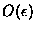terms, comprise first-order theory. Second-order theory includes the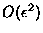terms.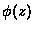and the linear long-wave propagation speed c are given by the eigenvalue problem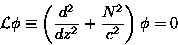(4)

with boundary conditions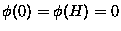. Only mode-1 waves are considered so c is the largest eigenvalue of (4) withthe corresponding eigenfunction. The Ei,jq can be expressed in terms of the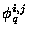which are determined by equations of the form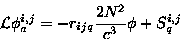(5)

where Si,jq is a known function of the lower-order vertical structure functions. The general solution includes an undetermined constant multiple of(see 3). The constants rijq are determined by the solvability conditions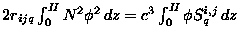. The first-order constants r10 and r01are given by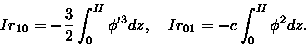(6)

where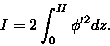(7)

The second order constants are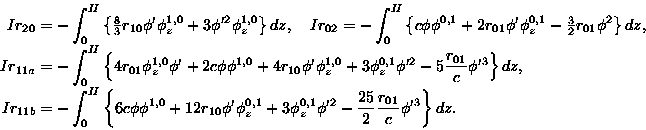(8)

The theory also yields an evolution equation for B(x,t), namely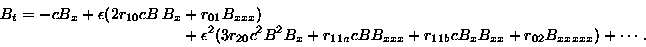(9)

Tothis is the KdV equation.

Euler-Lagrange Formulation

To use the Lagrangian coordinateas the independent variable instead of z we must be able to solve z = z(x,y,t) for y. This requires that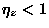and in particular rules out waves with overturning isopycnals. The governing equations (1a)-(1b) are replaced by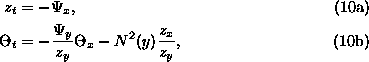where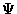and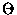are the streamfunctionand vorticity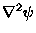expressed as functions of (x,y,t).

Solutions of (10a)-(10b) have the form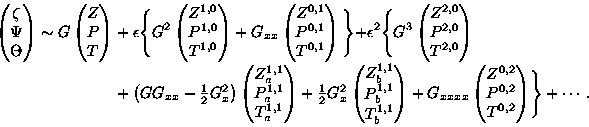(11)

where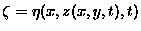is the isopycnal displacement written as a function of yG = G(x,t), and Z, P and T with the various indices are functions of y. G(x,t) satisfies (9) with identical values for the coefficients. Z andsatisfy the same eigenvalue problem so, if they are both scaled to have a maximum value of 1, they are identical. In that case we can take B=G. The remaining vertical structure functions can be expressed in terms of the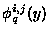Comparisons with fully-nonlinear waves

The theoretical approximations are now compared with fully nonlinear waves. Two different density profiles are considered in a fluid of depth H=100 m. The first,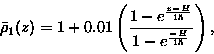(12)

has an exponentially increasing stratification. The second,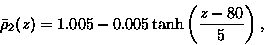(13)

has a well defined pycnocline 20 m below the surface. The bottom to surface density difference is 0.01 in both cases. For both stratifications the wave half-widths are approximately 100 m for the largest waves. Comparisons of the isopycnal displacement along the vertical line through the centre of a number of waves are shown in figures 1 and 2. Solid curves are profiles from the fully nonlinear waves. Dashed curves are the theoretical profiles. In the Euler-Lagrange formulation the leading order isopycnal displacement is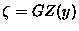. For the Eulerian formulation the isopycnal displacement is obtained by expanding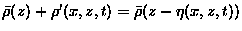which gives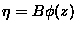at leading-order. Since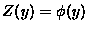both expression have the identical form however there is a crucial difference between the two. In the Eulerian expression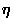is the vertical displacement of an isopycnal as a function of the displaced isopycnal height. In the Euler-Lagrange expression the vertical displacement of an isopycnal is given as a function of the rest height of the isopycnal. In figure 1 it can be seen that for density 1 the Eulerian description is much better than the Euler-Lagrange description while figure 2 shows that the opposite is true for density 2. Using first-order theory the theoretical isopycnal profiles obtained using the Eulerian formulation are almost indistinguishable from the fully-nonlinear wave profiles. This is remarkable as the largest wave considered is very close to breaking (i.e.,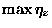is close to 1). Use of first-order theory for density 2 also reduces the error significantly but the agreement is not as good. For density 2 the largest wave is far from breaking.

In figures 3 and 4 vertical profiles of the horizontal velocity through the centre of the waves are shown. Solid curves are the profiles for the fully-nonlinear waves. Dashed profiles are the theoretical results. In figure 3 leading- and first-order Eulerian approximations are shown for density 1. In figure 4 leading- and first-order Euler Lagrange approximations are shown for density 2. For the Eulerian formulation the first-order velocity is given by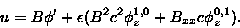(14)

For the Euler-Lagrange formulation u is given, at first-order, by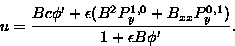(15)

For leading-order theory theterms are dropped. For the first-order expressions for u the value of Bxx is needed in the centre of the wave. For this the well-known solitary wave solution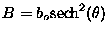of the KdV equation is used to express Bxx(0,0) in terms of bo. Here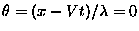at the wave centre. For the vertical profiles the second-order coefficients r20, r11a, r11b and r02 are not required, however for horizontal profiles they are required to calculatecorrections to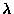and to the expression for B.

For density 1 the theoretical estimate is very good for even the largest wave. For density 2 the magnitude of the velocities is underestimated for the larger waves well below the pycnocline. The theory does correctly predict a minimum in uimmediately below the pycnocline, something that is not predicted by leading-order theory. The Eulerian formulation incorrectly predicts a subsurface maximum in u for the largest 2 waves (not shown).

These results show that the best formulation of shallow-water weakly-nonlinear theory for solitary internal waves is stratification dependent. Comparisons for more complicated stratifications taken from oceanographic observations will be shown.

References

(1) Turkington, B., Eydeland, A., and S. Wang, Stud. Appl. Math., 85, 93-127, 1991.

(2) Benney, D. J., J. Math. Phys., 45, 52-63, 1966.

(3) Lamb, K. G., and L. Yan, J. Phys. Oceanogr., 26, 2712-2734, 1996.

(4) Gear, J. A., and R. Grimshaw, Phys. Fluids. 26, 14-29, 1983.

• Figures ...

•Next:Figures ...

1998-09-17